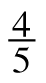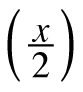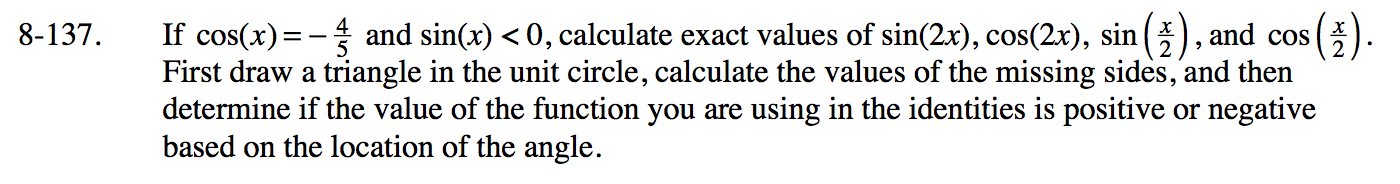### Home > PC3 > Chapter 8 > Lesson 8.3.4 > Problem8-137

8-137.

If cos(x) = –and sin(x) < 0, calculate exact values of sin(2x), cos(2x), sin, and cos. First draw a triangle in the unit circle, calculate the values of the missing sides, and then determine if the value of the function you are using in the identities is positive or negative based on the location of the angle. Homework Help ✎Double-Angle Formulas
sin(2α) = 2sin(α)cos(α)
cos(2α) = cos2(α) − sin2(α)

Half-Angle Formulas

$\sin\left ( \frac{\theta }{2} \right )=\pm \sqrt{\frac{1-\cos(\theta)}{2}}$

$\cos\left(\frac{\theta }{2} \right )=\pm \sqrt{\frac{1+\cos(\theta)}{2}}$

Draw a unit circle and include a right triangle matching the given conditions.
Use the Pythagorean Theorem to determine the value of the missing leg.